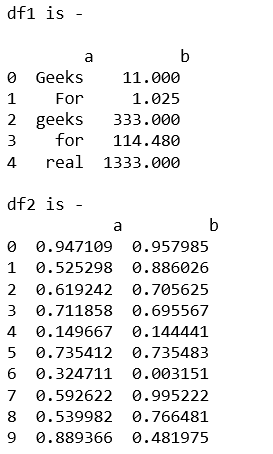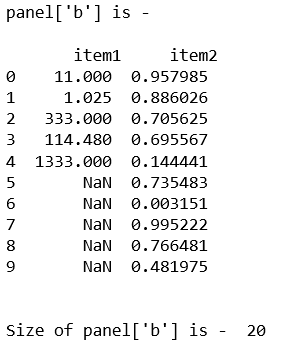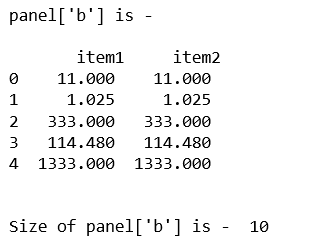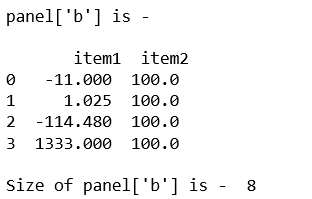GeeksforGeeks App
Open AppBrowser
Continue

# Python | Pandas Panel.size

In Pandas, Panel is a very important container for three-dimensional data. The names for the 3 axes are intended to give some semantic meaning to describing operations involving panel data and, in particular, econometric analysis of panel data.

In Pandas` Panel.size` gives the number of rows if Series. Otherwise return the number of rows times number of columns if DataFrame.

Syntax: Panel.size

Parameters: None

Returns: Returns value in int representing the number of elements in this object.

Code #1:

 `# importing pandas module  ``import` `pandas as pd  ``import` `numpy as np ``   ` `df1 ``=` `pd.DataFrame({``'a'``: [``'Geeks'``, ``'For'``, ``'geeks'``, ``'for'``, ``'real'``],  ``                    ``'b'``: [``11``, ``1.025``, ``333``, ``114.48``, ``1333``]}) ``                     ` `print``(``"df1 is - \n\n"``, df1)`` ` `# Create a 5 * 5 dataframe ``df2 ``=` `pd.DataFrame(np.random.rand(``10``, ``2``), columns ``=``[``'a'``, ``'b'``]) ``print``(``"df2 is - \n\n"``, df2)`Now, let’s create the Panel using dict of df1 and df2, and get the size of that panel.

 `data ``=` `{``'item1'``:df1, ``'item2'``:df2} ``   ` `# creating Panel  ``panel ``=` `pd.Panel.from_dict(data, orient ``=``'minor'``) ``print``(``"panel['b'] is - \n\n"``, panel[``'b'``], ``'\n'``) `` ` ` ` `print``(``"\nSize of panel['b'] is - "``, panel[``'b'``].size) `

Output:Code #2:

 `# importing pandas module  ``import` `pandas as pd  ``import` `numpy as np ``   ` `df1 ``=` `pd.DataFrame({``'a'``: [``'Geeks'``, ``'For'``, ``'geeks'``, ``'for'``, ``'real'``],  ``                    ``'b'``: [``11``, ``1.025``, ``333``, ``114.48``, ``1333``]}) ``                       ` `data ``=` `{``'item1'``:df1, ``'item2'``:df1} ``   ` `# creating Panel  ``panel ``=` `pd.Panel.from_dict(data, orient ``=``'minor'``) ``   ` `print``(``"panel['b'] is - \n\n"``, panel[``'b'``], ``'\n'``) ``   ` `print``(``"\nSize of panel['b'] is - "``, panel[``'b'``].size)   `

Output:Code #3:

 `# importing pandas module ``import` `pandas as pd ``import` `numpy as np `` ` `df1 ``=` `pd.DataFrame({``'a'``: [``'Geeks'``, ``'For'``, ``'geeks'``, ``'real'``], ``                    ``'b'``: [``-``11``, ``+``1.025``, ``-``114.48``, ``1333``]}) `` ` `df2 ``=` `pd.DataFrame({``'a'``: [``'I'``, ``'am'``, ``'dataframe'``, ``'two'``], ``                    ``'b'``: [``100``, ``100``, ``100``, ``100``]}) ``                     ` `data ``=` `{``'item1'``:df1, ``'item2'``:df2}`` ` `# creating Panel ``panel ``=` `pd.Panel.from_dict(data, orient ``=``'minor'``) ``print``(``"panel['b'] is - \n\n"``, panel[``'b'``]) `` ` `print``(``"\nSize of panel['b'] is - "``,  panel[``'b'``].size) `

Output:My Personal Notes arrow_drop_up